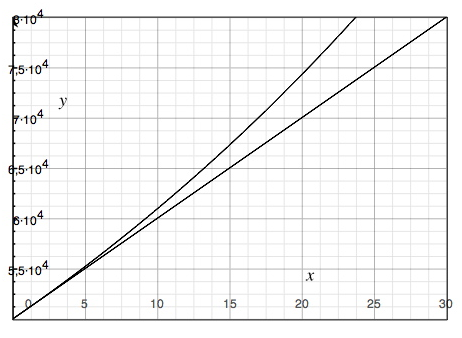# Exponential growth functions

We have dealt with linear functions earlier. All types of equations containing two unknown (x and y) variables may be inserted in a coordinate system. These types of equations are known as functions. A straight line is known as a linear function.

The function need not necessarily respond like a straight line equation. For example: If we have \$50 000 deposited in the bank, and receive a 2 % interest annually, our investment shall increase as follows:

 Year Capital Interest Sum 1 50 000 50 000 · 0.02 = 1 000 51 000 2 51 000 51 000 · 0.02 = 1 020 52 020 3 52 020 52 020 · 0.02 = 1040.40 53 060.40

Compare that with what we would have with a linear increase (2%):

 Year Capital Increase Sum 1 50 000 50 000 · 0.02 = 1000 51 000 2 51 000 50 000 · 0.02 = 1000 52 000 3 52 000 50 000 · 0.02 = 1000 53 000

In this case we may note that the increase was constant each year. The investment may be described as:

$y=50000+1000x$

where x equals the number of years.

However in the first case, the structure proceeds as:

$y=5000\cdot 1.02^{x}$

$investment \:\: after \: x \: number \: of\: years$

$= initial\: capital \cdot compound\: interest^{x \: number \: of \: years}$

Here we have an x-variable in the exponent. The interest and thus also the function are exponentials.

Now we shall examine the differences displayed with the functions in our example above in a coordinate system.The lower straight line represents the linear increase and the upper bowed curve represents the exponential increase. In other words it is more profitable to have a compounded interest than a fixed return.

An exponential function is a nonlinear function that has the form of

$y=ab^{x},\: \: where\: a\neq 0,b> 0$

An exponential function with a > 0 and b > 1, like the one above, represents an exponential growth and the graph of an exponential growth function rises from left to right.

An exponential function where a > 0 and 0 < b < 1 represents an exponential decay and the graph of an exponential decay function falls from left to right.

When a quantity increases or decreases exponentially it increases or decreases by the same percent over equal time periods in comparison to when a compound increases or decreases linearly when a quantity increases or decreases with the same amount over equal time periods.

## Video lesson

Graph the functions

$y_{1}=3^{x}$

$y_{2}=3x$